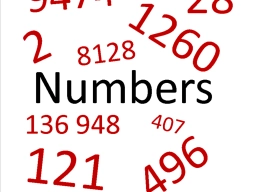# Multiple numerical bases

Find the value of x for which 312four+52x=96ten . Hint: four, x, and ten are the base of the given number.

x =  8

### Step-by-step explanation:Did you find an error or inaccuracy? Feel free to write us. Thank you!

Tips for related online calculators
Do you have a linear equation or system of equations and looking for its solution? Or do you have a quadratic equation?